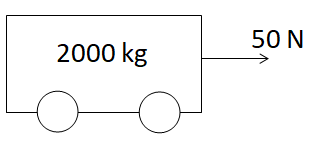A machine gun is mounted on a 2000 kg car on a horizontal frictionless surface. At some instant the gun fires bullets of mass 10 gm with a velocity of 500 m/sec with respect to the car. The number of bullets fired per second is ten. The average thrust on the system is

(1) 550 N

(2) 50 N

(3) 250 N

(4) 250 dyne

Concept Questions :-

Newton laws
High Yielding Test Series + Question Bank - NEET 2020

Difficulty Level:In the above diagram, the acceleration of the car will be

(1) 0.25 m/sec2

(2) 2.5 m/sec2

(3) 5.0 m/sec2

(4) 0.025 m/sec2

Concept Questions :-

Application of laws
High Yielding Test Series + Question Bank - NEET 2020

Difficulty Level:

A particle of mass 0.3 kg is subjected to a force F = –kx with k = 15 N/m. What will be its initial acceleration if it is released from a point 20 cm away from the origin

(1) 5 m/s2

(2) 10 m/s2

(3) 3 m/s2

(4) 15 m/s2

Concept Questions :-

Spring force
High Yielding Test Series + Question Bank - NEET 2020

Difficulty Level:

An elevator weighing 6000 kg is pulled upward by a cable with an acceleration of 5 ms–2. Taking g to be 10 ms–2, then the tension in the cable is

(1) 6000 N

(2) 9000 N

(3) 60000 N

(4) 90000 N

Concept Questions :-

Application of laws
High Yielding Test Series + Question Bank - NEET 2020

Difficulty Level:

A ball of mass 0.2 kg moves with a velocity of 20 m/sec and it stops in 0.1 sec; then the force on the ball is

(1) 40 N

(2) 20 N

(3) 4 N

(4) 2 N

Concept Questions :-

Application of laws
High Yielding Test Series + Question Bank - NEET 2020

Difficulty Level:

The ratio of the weight of a man in a stationary lift and when it is moving downward with uniform acceleration ‘a’ is 3 : 2. The value of ‘a’ is (g-Acceleration due to gravity of the earth)

(1) $\frac{3}{2}g$

(2) $\frac{g}{3}$

(3) $\frac{2}{3}g$

(4) g

Concept Questions :-

Application of laws
High Yielding Test Series + Question Bank - NEET 2020

Difficulty Level:

If force on a rocket having exhaust velocity of 300 m/sec is 210 N, then rate of combustion of the fuel is

(1) 0.7 kg/s

(2) 1.4 kg/s

(3) 0.07 kg/s

(4) 10.7 kg/s

Concept Questions :-

Variable mass system
High Yielding Test Series + Question Bank - NEET 2020

Difficulty Level:

In an elevator moving vertically up with an acceleration g, the force exerted on the floor by a passenger of mass M is

(1) Mg

(2) $\frac{1}{2}Mg$

(3) Zero

(4) 2 Mg

Concept Questions :-

Application of laws
High Yielding Test Series + Question Bank - NEET 2020

Difficulty Level:

A 5000 kg rocket is set for vertical firing. The exhaust speed is 800 ms–1. To give an initial upward acceleration of 20 ms–2, the amount of gas ejected per second to supply the needed thrust will be (g = 10 ms–2)

(1) 127.5 kg s–1

(2) 187.5 kg s–1

(3) 185.5 kg s–1

(4) 137.5 kg s–1

Concept Questions :-

Variable mass system
High Yielding Test Series + Question Bank - NEET 2020

Difficulty Level:

If a person with a spring balance and a body hanging from it goes up and up  in an aeroplane, then the reading of the weight of the body as indicated by the spring balance will

(1) Go on increasing

(2) Go on decreasing

(3) First increase and then decrease

(4) Remain the same

Concept Questions :-

Spring force